Next: Charge of a Klein-Gordon Up: Klein-Gordon Equation Previous: General Solution of the

# Conserved Current and Charge

Now that we have found the general solution to the Klein-Gordon equation, we look for a physical interpretation of the wave function. Following the approach used in nonrelativistic quantum mechanics, we define the position probability density,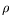, and current probability density,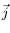, which are required to satisfying the continuity equation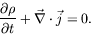(4.31)

Using the four-vector notation,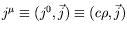, we can write the continuity equation in the more compact form: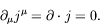(4.32)

Integrating the position probability density over a volume,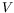, large enough to contain all the probability, and using the divergence theorem, we write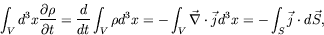(4.33)

where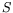is the surface which bounds the volume. We see that any probability which flows out of the volumemust pass through the surface. The continuity equation (4.31) implies that probability cannot be created or destroyed at any point; it can only flow from one point to another. Thus probability that satisfies the continuity equation will be locally conserved.

We must now show that we can construct a position probability density and current probability density which satisfies a continuity equation. Multiplying the Klein-Gordon equation for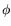on the left by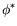, we write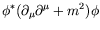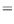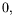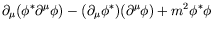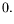(4.34)

Similarly, multiplying the Klein-Gordon equation foron the left by, we write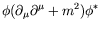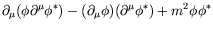(4.35)

Subtracting equation 4.34 from 4.35 gives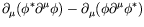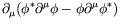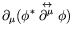(4.36)

The resulting conserved four-current,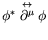, can be seen to be purely imaginary by taking it complex conjugate.

We want to interpret the zero component of the conserved four-current as the probability density, so we multiply it by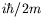. This normalization makes the current real and have dimensions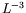. The normalization also causes the three-current probability density to have the same form as in nonrelativistic quantum mechanics. Applying this normalization, we write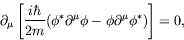(4.37)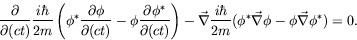(4.38)

We define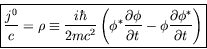(4.39)

and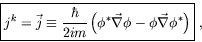(4.40)

such thatandsatisfy the continuity equation (4.32). The expression foris identical with the nonrelativistic form and the expression forcan be shown to reduce to the nonrelativistic form in the nonrelativistic limit.

We wanted to interpretas a position probability density, but it is not positive definite. The probability density'' now contains time derivatives since the Klein-Gordon equation is second order in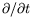. This means thatis not constrained to be positive definite since at a given time bothand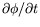may have arbitrary values. Thereforemay be either positive or negative.

To circumvent the problem of a non-positive definite current density, we need a wave equation first-order in the time derivative. The Dirac equation, developed in chapter 5, is first-order in the time derivative but we will find that it still proves impossible to retain a positive definite probability density for a single particle, while at the same time providing a physical interpretation of the negative-energy solution. This means that the Klein-Gordon equation is no worse than the Dirac equation with respect to physically interpreting the probability density for a single particle.

As an example, let us calculate the current four-vector using plane waves and setting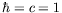. We obtain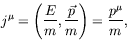(4.41)

where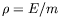can be either positive or negative, depending on the sign of the energy.

In obtaining equation 4.41, we have used un-normalized plane waves. Often the exponential of the plane-wave solutions is multiplied by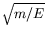so that the time component of the current is normalized to unity.

The form of the current density in equation 4.41 is as expected. To see this, we consider a volume of space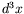in the rest frame containing charge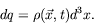(4.42)

Then in the primed frame moving with a velocity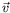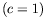, the corresponding volume is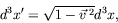(4.43)

since the dimension of the volume in the direction of the boast is reduced by Lorentz contraction. The same amount of charge must be contained in this Lorentz contracted volume, so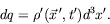(4.44)

We then find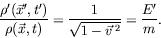(4.45)

Also, we expect the spatial current density to be given by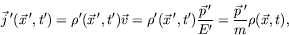(4.46)

or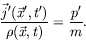(4.47)

Equations 4.45 and 4.47 lead us to interpret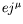as the current density four-vector, where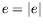is the fundamental electric charge, i.e.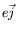is the electronic charge density. The continuity equation thus becomes a statement of the conservation of charge, assuming that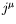has been normalized to be real. As we will see, for a theory representing reality, the number of particles many not be conserved and thus the interpretation ofas a current density only applies to a single-particle (single-charge) theory.

Let us return to the continuity equation and the divergence theorem. Integration of the current over the configuration space gives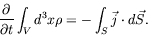(4.48)

If we now take our volume of integration arbitrarily large, and if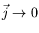as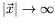sufficiently rapidly, then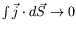on the surface. This is required of a physical current. Thus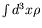is constant in time. We interpret this constant as the electric charge,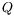, of the solution to the Klein-Gordon equation and define it using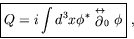(4.49)

where the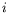makespurely real.

SubsectionsNext: Charge of a Klein-Gordon Up: Klein-Gordon Equation Previous: General Solution of the
Douglas M. Gingrich (gingrich@ ualberta.ca)
2004-03-18